Home > CCA2 > Chapter 8 > Lesson 8.3.3 > Problem8-172

8-172.
1. Decide which of the following equations have real roots, and which have complex roots without completely solving them. Homework Help ✎

1. y = x2 − 6

2. y = x2 + 6

3. y = x2 − 2x + 10

4. y = x2 − 2x − 10

5. y = (x − 3)2 − 4

6. y = (x − 3)2 + 4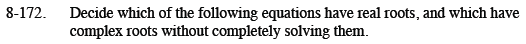Think about the graph of each given function. Are there any x-intercepts?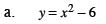real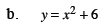complex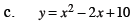complex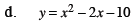real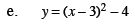real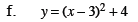complex